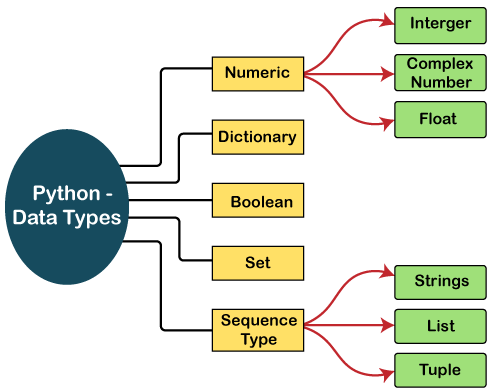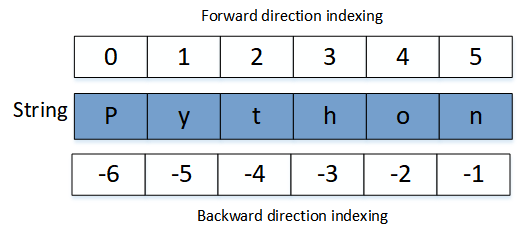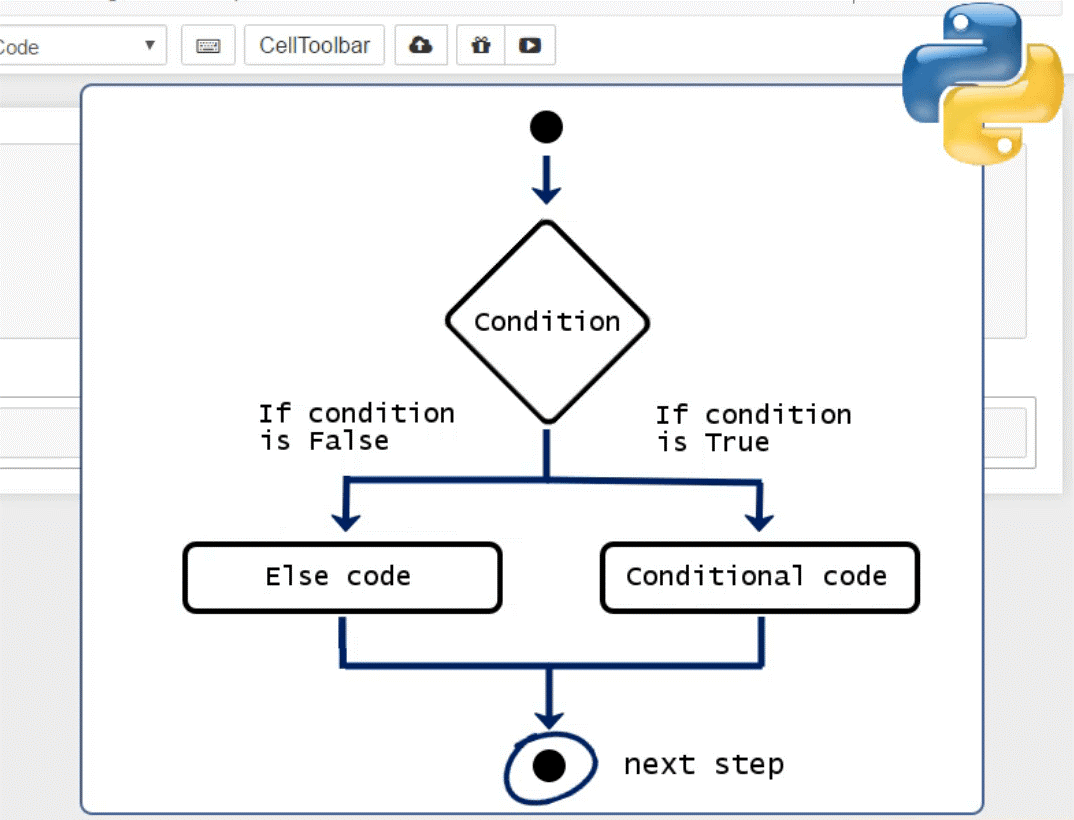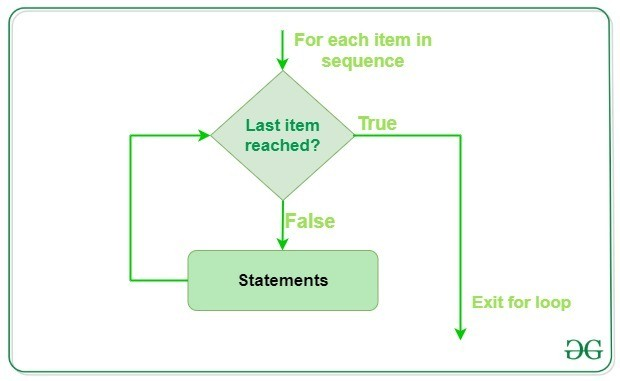As you know with the growth in Machine Learning & Artificial Intelligence, the future of the industry will be dependent on AI-based applications. Python is the stepping stone to start your career in automation and data science.

Few real-time application of Python:

1. Web Scraping
2. Image Processing
3. Machine Learning & AI
4. Automate excel & CSV files
5. Scientific & Numeric Computing
6. Email Automation
7. Data Analysis
8. Data Visualization
9. Web Development using Django
10. Gaming development

Benefits of using Python over other programming languages:

• Open Source (No license required to buy software) & supports Community development
• Extensively Support multiple Libraries
• Data Analysis is done using Pandas
• Numerical Calculation is done using NumPy
• Object-Oriented programming language
• Python is easier than C, C++, Java, .Net, etc.
• High-performance speed
• Better productivity
• User-friendly data-structures

This tutorial will cover:-

1. Python Installation
2. Python Data Types
• Numbers
• Strings
• List
• Tuple
• Dictionary
• Sets
1. Operators
2. Conditionals Statements
3. Loops
4. Functions
5. File Handling

## How to install Python

2. Complete setup installation
3. Install visual studio code from code.visualstudio.com
4. Start using Jupyter notebook

Variables

Python stores data/ values in memory using a variable. A variable is a name given by you, to which you assign a piece of data that is stored in an area of the computer’s memory, allowing you to refer to that data when you need to later in the program

a = 10

b = 'Welcome to Gyansetu!'

c = 10.11

print(a,b,c)

## output:

## 10 Welcome to Gyansetu! 10.11

Here the variables are a, b, c

## Python Data TypesNumbers:

There are 3 types of Numbers:

1. Integer
2. Float
3. Complex
1. Integer (int): Integer is a non-decimal number formed by the combination of 0 – 9 digits.
2. Float (float): A float is a decimal number that can be represented on a number line.
3. Complex (complex): They are the numbers consist of an imaginary number and a real number.

Different types of numbers

`a = 10   #integer`

b = 43.49     #float

c = 1 + 2j       #complex

To accept values from user input

``````x = input("Enter any number : ")

y = input("Enter any number : ")

z = input("Enter any number : ")

print(x,y,z)```

## output:

## Enter any number : 2

## Enter any number : 3

## Enter any number : 1

## 2 3 1```

To know the type of data:

``````print(type(x))

print(type(y))

print(type(z))
```

## output:

##

##

## ```

String

A string in Python consists of a series or sequence of characters – letters, numbers, and special characters.

Strings can be indexed – often synonymously called subscripted as well. Similar to C, the first character of a string has the index 0.

How to print a string

```a = "sahil"

b = 20

c = 30

print("%d-%d-%d" % (a,b,c))

## output:

## This will give error because %d is used in print for a and a is a string, %d is used for integer values```

### Another way to solve the error

```a = "sahil"

b = 20

c = 30

print("%s-%d-%d" % (a,b,c))

## output:

## sahil-20-30

## %s is used for string values```

How to print a string using .format

```a = "sahil"

b = 20

c = 30

print("{}-{}-{}".format(a,b,c))

## output:

## sahil-20-30```

Indexing in Python:

Indexing # starts from 0 to n – 1 where n is the length of string use []

Example to understand indexing:```s = "Hello World!"

print(s)

print(s)

## output:

## l

## s```

Slicing in Python:

Slicing # used to make sub-strings from strings used with start, end, and step variable

```# s[start:end:step]

s = "Hello World!"

x = s[3:11]

y =  s[:]

z = s[:6]

p = s[6:]

print(x)

print(y)

print(z)

print(p)

print(s)

## output:

## lo World

## Hello World!

## Hello

## World!

## Hello World!```

Basic String Functions: –

swapcase, upper, lower, strip, lstrip, rstrip, find, replace, format, center

Swapcase:- used to change uppercase letters into lowercase letters and vice versa

Upper:- It changes all letters into uppercase and returns the string

Lower:- It changes all letters into lowercase and return the string

Strip:- strip is used to remove all leading and trailing spaces from the string

Lstrip:- It is used to remove left space

Rstrip:- It is used to remove the right space

Example to understand string functions:

```s = input("Enter a String : ")

print(s)

print("Swapcase : ",s.swapcase())

print("Upper : ",s.upper())

print("Lower : ",s.lower())

print("Strip : ",s.strip())

print("LStrip : ",s.lstrip())

print("RStrip : ",s.rstrip())

## output:

## Enter a String : iclass gyansetu

## iclass gyansetu

## Swapcase :  ICLASS GYANSETU

## Upper :  ICLASS GYANSETU

## Lower :  iclass gyansetu

## Strip :  iclass gyansetu

## LStrip :  iclass gyansetu

## RStrip :  iclass gyansetu```

Lists

• Lists are one of the most powerful tools in Python.
• They are just like the arrays declared in other languages.
• But the most powerful thing is that list need not be always homogeneous.
• A single list can contain strings, integers, as well as objects.
• Lists can also be used for implementing stacks and queues.
• Lists are mutable, i.e., they can be altered once declared.

Declaring a list:

```L = [1, "a" , "string" , 1+2]

print(L)

## output : [1, 'a', 'string', 3]```

Homogeneous & Non-Homogeneous List

Homogeneous List contains all elements of same data type.

Non- Homogeneous List contains elements of different data type.

```l = [ 'hello','hi','how are you' ]

print(l)

print(l)

l = [ 56,23,45,12,67,12,43,1,6,8,6,33,12]

print("Homogeneous List :",l)

print(l)

l = [ 'hello','hi', 3, 4.5 ]

print("Non-Homogeneous List : ",l)

print(l[-3])

## output:

## ['hello', 'hi', 'how are you']

## how are you

## Homogeneous List : [56, 23, 45, 12, 67, 12, 43, 1, 6, 8, 6, 33, 12]

## 12

## Non-Homogeneous List :  ['hello', 'hi', 3, 4.5]

## hi```

Tuple

• A Tuple is a collection of Python objects separated by commas.
• In someway a tuple is similar to a list in terms of indexing, nested objects, and repetition but a tuple is immutable, unlike lists which are mutable.

Declaring a Tuple

```tup = 'python', 'gyansetu'

print(tup)

## output: ('python', 'gyansetu')```
```### Another way for doing the same

tup = ('python', 'gyansetu')

print(tup)

## output: ('python', 'gyansetu')```

Dictionary

• It consists of key-value pairs.
• The value can be accessed by a unique key in the dictionary.

Dictionary Example with Key-Value pair

```mydict = {

'name':'python',

'build_year':1991,

'Father of Python':"Guido Van Rossum",

'versions' : [1.0,2.0,3.0],

'latest_version':3.6}

print(mydict)

print("Name : ", mydict['name']) #returns the value of the key 'name'

print("Frame Works : ", mydict['Frame_works'])

## output:

## {'name': 'python', 'build_year': 1991, 'Father of Python': 'Guido Van Rossum', 'Frame_works': ['Django', 'Flask', 'Web2PY', 'Torando', 'kivi'], 'versions': [1.0, 2.0, 3.0], 'latest_version': 3.6}

## Name :  python

## Frame Works :  ['Django', 'Flask', 'Web2PY', 'Torando', 'kivi']
```

## Operators in Python

###Arithmetic Operators

Arithmetic operators are used to performing mathematical operations like addition, subtraction, multiplication, and division

1. Addition Operator ( + )
2. Subtraction Operator ( – )
3. Multiplication Operator ( * )
4. Division Operator ( / )
5. Modulas Operator ( % )
6. Floor Division ( // )
7. Exponent Operator ( ** )

Example of Arithmetic Operator

```x = 10

y = 3

z1 = x+y

z2 = x-y

z3 = x * y

print("Addition of {} and {} is {}.".format(x,y,z1))

print("Subtraction of {} and {} is {}.".format(x,y,z2))

print("Multiplication of {} and {} is {}.".format(x,y,z3))

## output:

## Addition of 10 and 3 is 13.

## Subtraction of 10 and 3 is 7.

## Multiplication of 10 and 3 is 30.```

Comparison Operators

These operators compare the values on either side of them and decide the relation among them.

1. Less Than ( < )
2. Less Than Equals To ( <= )
3. Greater Than ( > )
4. Greater Than Equals To ( >= )
5. Equals To Equals To ( == )
6. Not Equals To ( != )

Example of Comparison Operator

```#returns true if statement is True else returns False

print("6 < 8 ", 6 < 8)               ## output: True

print("6 <= 6 ", 6 <= 6)         ## output: True

print("5 > 6 ", 5 > 6)              ## output: False

print("6 != 7 ", 6 != 7)           ## output: True```

Logical Operators

Logical operators are used on conditional statements (either True or False). They perform Logical AND, Logical OR, and Logical NOT operations

1. And

If x is false, return x

else return y

1. Or

If x is false, return y

else x

Examples:

```print( 5>7 and 6-5*3+6)                ## output: False

print( 6-4*3+6 and 5>7)                ## output: 0

print( 6-4*3+6 or 6 > 5)                  ## output: True

print( 6 > 5 or 6-4*3+6)                  ## output: True```

Membership Operator

Membership operators are operators used to validate the membership of a value. It test for membership in a sequence, such as strings, lists, or tuples.

1. in
2. not in

Examples:

```s2 = "Dog is an animal."

s1 = "Dog"

x = s1 in s2

if x :

print("Pattern Found in step 1") #return this if x=True

else :

p = s1 not in s2

print(x)

print(p)

## OUTPUT:

## Pattern Found in step 1

## True

## False

```

Identity Operator

Python Membership and Identity Operators · in operator: The ‘in’ operator is used to check if a value exists in a sequence or not.

1. is
2. is not

Examples:

```x = 5

y = 5

print(x is y)

print( x is not y )

p = 3

q = 4

if p is q :

print("Both are equal")

else :

print("Both are different")```

## Conditional Statements

In programming and scripting languages, conditional statements or conditional constructs are used to perform different computations or actions depending on whether a condition evaluates to true or false. (Please note that true and false are always written as True and False in Python.)

### Example to calculate the greatest of 3 numbers using a conditional statement

```#Greatest among Three numbers

a = int(input("A : "))

b = int(input("B : "))

c = int(input("C : "))

if a >= b :

if a >= c :

print("A is greatest ")

else :

print("C is greatest ")

elif b >= c :

print("B is Greatest")

else :

print("C is Greatest ")

## OUTPUT:

## A : 12

## B : 32

## C : 16

## B is Greatest```

## Loops in Python

Loops can be divided into 2 kinds.

• Finite: This kind of loop works until a certain condition is met
• Infinite: This kind of loop works infinitely and does not stop ever.There are 2 kinds of loops:

• for
• while

For Loops: These loops are used to perform a certain set of statements for a given condition and continue until the condition has failed. You know the number of times that you need to execute the for loop.

Example of For loop

```print("List Iteration")

l = ["books", "bags", "pens"]

for i in l:

print(i)

## OUTPUT:

## List Iteration

## books

## bags

## pens```

For loop using range()

```for i in range(5):

print(i)

## OUTPUT:

## 0

## 1

## 2

## 3

## 4

```

If you want to explore and learn coding skills in Python, then Gyansetu provides you the best Python Training Course or Call-  8130799520.